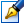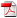##Qualitative study of cubic systems of differential equations with six and with seven real invariant straight lines

 Author: Puţuntică Vitalie Degree: doctor of physics and mathematics Speciality: 01.01.02 - Differential equations Year: 2010 Scientific adviser: Alexandru Şubădoctor habilitat, professor, Tiraspol State University Institution: Tiraspol State University Scientific council: DH 30-01.01.02-27.03.08 Moldova State University

### Status

The thesis was presented on the 29 April, 2010
Approved by NCAA on the 3 June, 2010

### Abstract0.62 Mb / in romanian

### Keywords

cubic system of differential equations, invariant algebraic curve, invariant exponential function, Darboux integrability, singular point, phase portrait.

### Summary

It was perfected in Chisinau, in 2010, it is written in Romanian and consists of an Introduction, 4 chapters, concluzions, bibliography of 111 titles, 123 pages of basic text, 63 figures and 24 tables. In the thesis topic 15 scientific papers are published.

The topic of thesis is The Qualitative Theory of Differential Equations. The subject of study is the system of differential equation
x=P(x,y), y=Q(x,y)

where x=P(x,y) and y=Q(x,y) are polynomials of degree three in x and y with real coefficients. The aim of the thesis is the study of cubic systems with invariant straight lines, having the total sum of degrees of invariance equal to six (seven). Its objectives are: determination of cubic system which have six (seven) invariant straight lines and further research of these systems.

In the thesis the cubic systems with six (seven) invariant straight lines (taking in account the degrees of invariance), canonical forms are constructed, the bifurcation diagrams in the space of coefficients were established, the phase portraits were constructed on the Poincare disc.

Results of thesis are new and represent a continuation in the study of cubic systems with invariant straight lines. They can be used in further investigations of cubic systems with invariant algebraic curves, in courses delivered at the university faculties of real profile; in the study of models which describe certain phenomena in physics, chemistry, biology, economy etc.